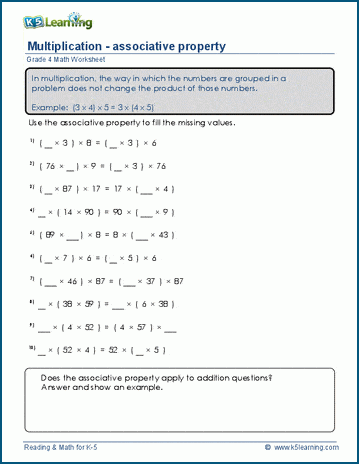# The Special Math Rule of Associative Property

## What is associative property?

The associative property is a special rule in math that helps us change the way we group numbers when we're adding or multiplying them. It says that no matter how we group the numbers, the final answer will still be the same.

For addition, it means that when we're adding three or more numbers, we can group them in different ways and still get the same total. Here's an example:

Let's say we have the numbers 2, 3, and 4. We want to add them together. Using the associative property, we can group them like this:

(2 + 3) + 4 = 5 + 4 = 9

Or we can group them like this:

(3 + 4) + 2 = 2 + 7 = 9

No matter how we group them, we still get the same answer, which is 9.

## Associative property for multiplication

For multiplication, it's the same idea. We can group the numbers differently, but the answer will still be the same. Here's an example:

Let's use the numbers 2, 4, and 5. We want to multiply them together. Using the associative property, we can group them like this:

(2 × 4) × 5 = 8 × 5 = 40

Or we can group them like this:

(4 × 5) x 2 = 2 × 20 = 40

Again, the answer is the same, which is 40.

## Practice associative property in multiplication

We have a series of worksheets in our grade 4 section that helps students practice grouping numbers in multiplication.Become a Member

This content is available to members only.